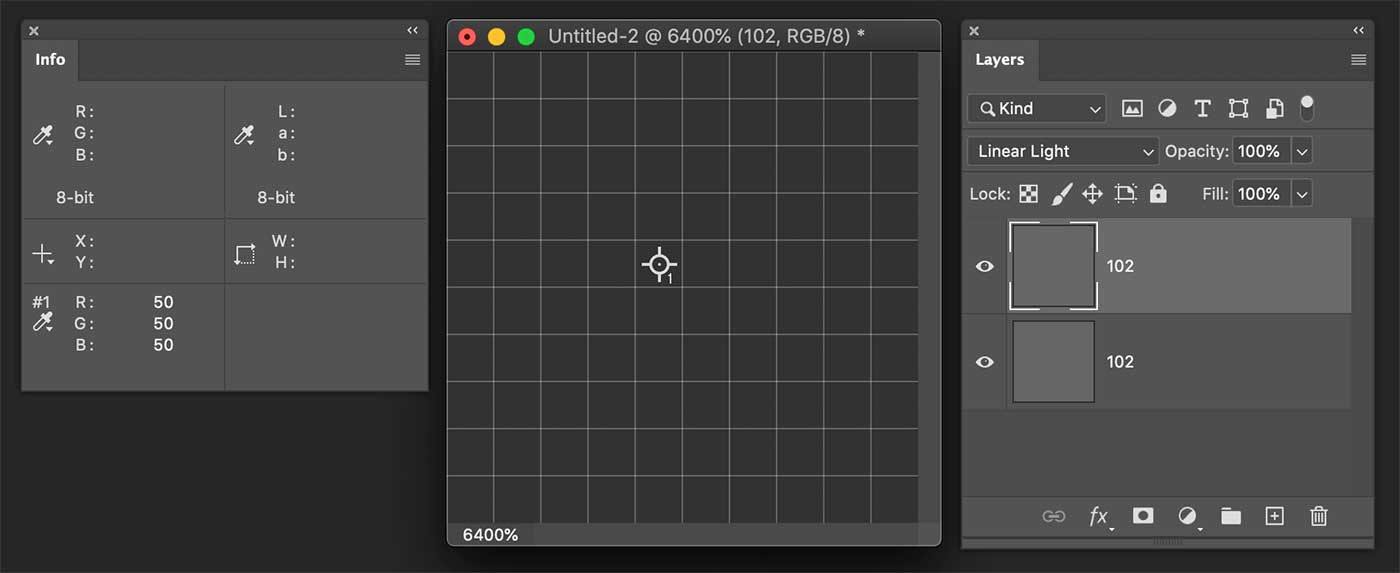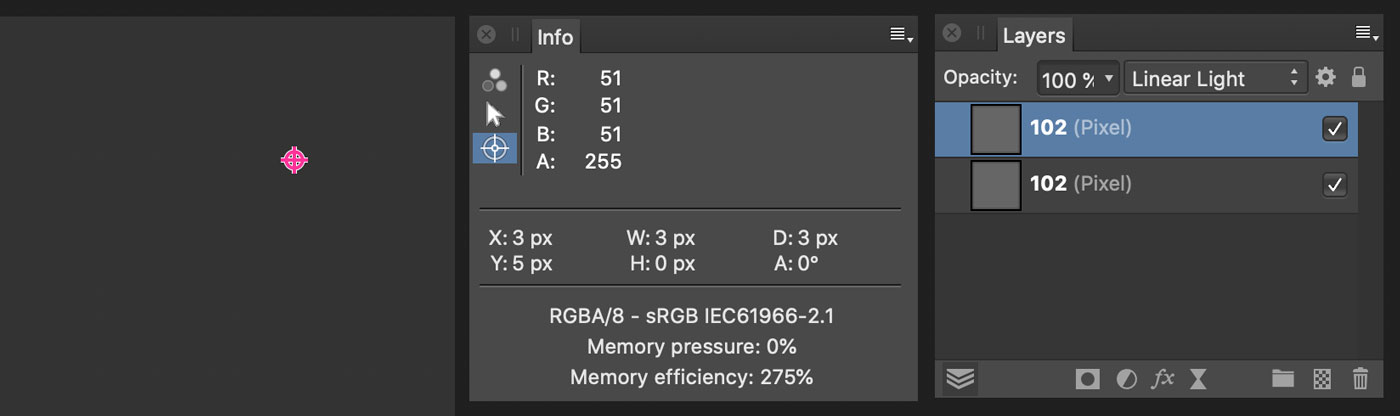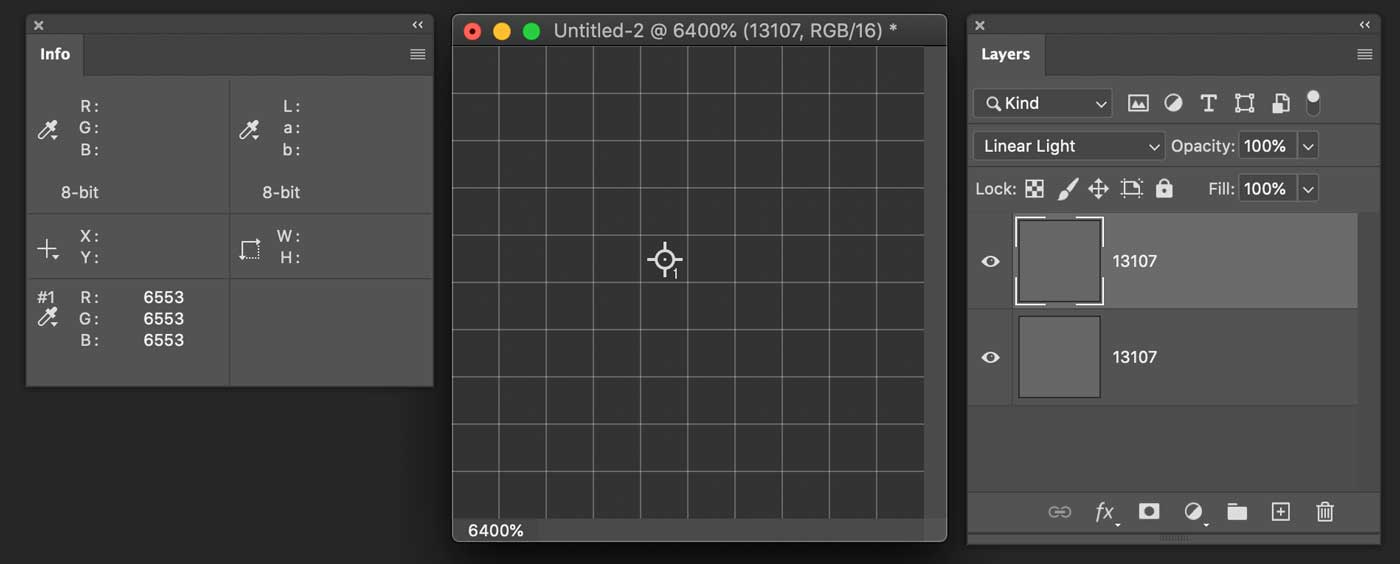##### davidebarranca23 Messages

•

744 Points

Mon, Feb 1, 2021 11:40 AM

# Linear Light 8bit math off by 1?

The Linear Light blending mode formula for a 2 layers setup with B as the layer below, and A as the active layer on top, for which the blending mode is set, is:

LL = 2A + B - 1

This for normalized values. Meaning that 1 should be as the max value in the available range, i.e. white.

For 8 bit we've got 256 possible values in the (0,255) range hence 1 == 255.

Given the simplest setup possible, in a RGB 8bit file with solid color values R=G=B:For simplicity I've set A = B.

LL = 2A + B - 1 = 2*102 + 102 - 255 = 51.

Problem is that PS says 50, as in the screenshot above.

I've tested this on a variety of different values, and the result is always off by one, as if PS was using 256 as the max value in the range, like:

2*102 + 102 - 256 = 50.

That would be an anomaly, wouldn't it? I've tested on Affinity Photo just out of curiosity and the result is different than Photoshop's and consistent with the use of 255 as the max value:Please note that if you use normalized values for the calculation, nothing changes:

LL  = (2*(102/255) + 102/255 - 1) *255 = 51

So Photoshop is still off by one.

If you test 16bit files, where 16bit is in fact 15bit+1, i.e. 32768 + 1 = 32769 in the (0,32768) range, Photoshop now correctly uses the max available value in the range, 32768, for the calculation:LL = 2*13107 + 13107 - 32768 = 6553.

So it appears that for some reason I don't get, PS uses the wrong max value in calculating the Linear Light blending mode. Is this possible, and if by design, why?

Thanks!

Accepted Solution

Employee

•

251 Messages

•

3.9K Points

8 m ago

You are right!  We do purposefully subtract 1. The reasoning is that, without subtracting that 1, there would be no neutral color.

We think of the formula is being

LL = B + delta(A)

where

delta(A) = 2 * A - 255

With this form, delta(127) = 2 * 127 - 255 = -1, and delta(128) = 2 * 128 - 255 = +1.

What that means is that no matter what pixel value you overlay, the result will always be different than the base color, and there is no neutral.

When we implemented this, our team decided that having the neutral color was important enough to sacrifice a bit of accuracy. So the form we use in the code is

delta(A) = 2 * A - 255 - 1

so that delta(128) = 2 * 128 - 255 - 1 = 0.

Thanks for replying @andrew_sender, much appreciated!

It definitely makes sense.

Since you have a solid grasp of these matters, can you shed some light on this question as well? It's driving me crazy.

(to frame all these bizarre issues I have: I'm working on an essay on frequency separation and I need to understand how calculations are made :-) )

Thanks!!

Davide